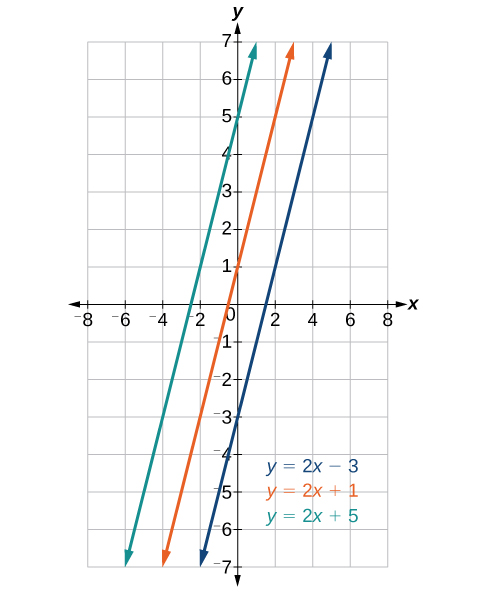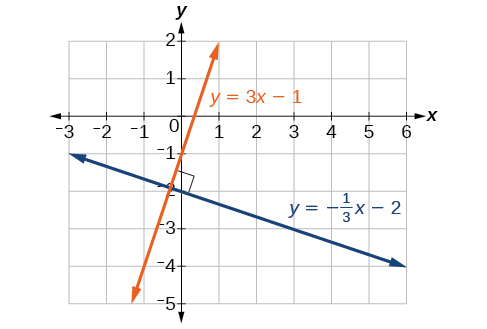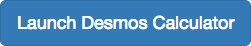## Parallel and Perpendicular Lines

### Learning Outcomes

• Determine whether two lines are parallel or perpendicular.
• Find the equations of parallel and perpendicular lines.
• Write the equations of lines that are parallel or perpendicular to a given line.

Parallel lines have the same slope and different y-intercepts. Lines that are parallel to each other will never intersect. For example, the figure below shows the graphs of various lines with the same slope, $m=2$.Parallel lines have slopes that are the same.

All of the lines shown in the graph are parallel because they have the same slope and different y-intercepts.

Lines that are perpendicular intersect to form a ${90}^{\circ }$ angle. The slope of one line is the negative reciprocal of the other. We can show that two lines are perpendicular if the product of the two slopes is $-1:{m}_{1}\cdot {m}_{2}=-1$. For example, the figure below shows the graph of two perpendicular lines. One line has a slope of 3; the other line has a slope of $-\frac{1}{3}$.

$\begin{array}{l}\text{ }{m}_{1}\cdot {m}_{2}=-1\hfill \\ \text{ }3\cdot \left(-\frac{1}{3}\right)=-1\hfill \end{array}$Perpendicular lines have slopes that are negative reciprocals of each other.

### Example: Graphing Two Equations, and Determining Whether the Lines are Parallel, Perpendicular, or Neither

Graph the equations of the given lines and state whether they are parallel, perpendicular, or neither: $3y=-4x+3$ and $3x - 4y=8$.

### Try It

Graph the two lines and determine whether they are parallel, perpendicular, or neither: $2y-x=10$ and $2y=x+4$.

If we know the equation of a line, we can use what we know about slope to write the equation of a line that is either parallel or perpendicular to the given line.

## Writing Equations of Parallel Lines

Suppose we are given the following equation:

$y=3x+1$

We know that the slope of the line formed by the function is 3. We also know that the y-intercept is (0, 1). Any other line with a slope of 3 will be parallel to $y=3x+1$. So all of the following lines will be parallel to the given line.

$\begin{array}{lll}y=3x+6\hfill & \\ y=3x+1\hfill & \\ y=3x+\frac{2}{3}\hfill \end{array}$

Suppose then we want to write the equation of a line that is parallel to $y=3x+6$ and passes through the point (1, 7). We already know that the slope is 3. We just need to determine which value for b will give the correct line. We can begin with point-slope form of a line and then rewrite it in slope-intercept form.

$\begin{array}{llll}y-{y}_{1}=m\left(x-{x}_{1}\right)\hfill & \\ y - 7=3\left(x - 1\right)\hfill & \\ y - 7=3x - 3\hfill & \\ \text{}y=3x+4\hfill \end{array}$

So $y=3x+4$ is parallel to $y=3x+1$ and passes through the point (1, 7).

### How To: Given the equation of a LINE, write the equation of a line parallel to the given line that passes through A given point

1. Find the slope of the line.
2. Substitute the given values into either point-slope form or slope-intercept form.
3. Simplify.

### Example: Finding a Line Parallel to a Given Line

Find a line parallel to the graph of $y=3x+6$ that passes through the point (3, 0).

## Writing Equations of Perpendicular Lines

We can use a very similar process to write the equation for a line perpendicular to a given line. Instead of using the same slope, however, we use the negative reciprocal of the given slope. Suppose we are given the following line:

$y=2x+4$

The slope of the line is 2 and its negative reciprocal is $-\frac{1}{2}$. Any function with a slope of $-\frac{1}{2}$ will be perpendicular to $y=2x+4$. So all of the following lines will be perpendicular to $y=2x+4$.

$\begin{array}{lll}y=-\frac{1}{2}x+4\hfill & \\ y=-\frac{1}{2}x+2\hfill & \\ y=-\frac{1}{2}x-\frac{1}{2}\hfill \end{array}$

As before, we can narrow down our choices for a particular perpendicular line if we know that it passes through a given point. Suppose then we want to write the equation of a line that is perpendicular to $y=2x+4$ and passes through the point (4, 0). We already know that the slope is $-\frac{1}{2}$. Now we can use the point to find the y-intercept by substituting the given values into slope-intercept form and solving for b.

$\begin{array}{lllll}y=mx+b\hfill & \\ 0=-\frac{1}{2}\left(4\right)+b\hfill & \\ 0=-2+b\hfill \\ 2=b\hfill & \\ b=2\hfill \end{array}$

The equation for the function with a slope of $-\frac{1}{2}$ and a y-intercept of 2 is $y=-\frac{1}{2}x+2$.

So $y=-\frac{1}{2}x+2$ is perpendicular to $y=2x+4$ and passes through the point (4, 0). Be aware that perpendicular lines may not look obviously perpendicular on a graphing calculator unless we use the square zoom feature.

### Q & A

A horizontal line has a slope of zero and a vertical line has an undefined slope. These two lines are perpendicular, but the product of their slopes is not –1. Doesn’t this fact contradict the definition of perpendicular lines?

No. For two perpendicular linear functions, the product of their slopes is –1. As you will learn later, a vertical line is not a function so the definition is not contradicted.

### How To: Given the equation of a LINE, write the equation of a line Perpendicular to the given line that passes through A given point

1. Find the slope of the given line.
2. Determine the negative reciprocal of the slope.
3. Substitute the slope and point into either point-slope form or slope-intercept form.
4. Simplify.

### Example: Finding the Equation of a Perpendicular Line

Find the equation of a line perpendicular to $y=3x+3$ that passes through the point (3, 0).

### Try It

Given the line $y=2x - 4$, write an equation for the line passing through (0, 0) that is

1. parallel to y
2. perpendicular to y

Check your work with an online graphing tool.### How To: Given two points on a line, write the equation of A perpendicular line that passes through A Third point

1. Determine the slope of the line passing through the points.
2. Find the negative reciprocal of the slope.
3. Substitute the slope and point into either point-slope form or slope-intercept form.
4. Simplify.

### Example: Finding the Equation of a Perpendicular Line

A line passes through the points (–2, 6) and (4, 5). Find the equation of a perpendicular line that passes through the point (4, 5).

### Try It

A line passes through the points (–2, –15) and (2, –3). Find the equation of a perpendicular line that passes through the point (6, 4).

## Writing the Equations of Lines Parallel or Perpendicular to a Given Line

As we have learned, determining whether two lines are parallel or perpendicular is a matter of finding the slopes. To write the equation of a line parallel or perpendicular to another line, we follow the same principles as we do for finding the equation of any line. After finding the slope, use point-slope form to write the equation of the new line.

### Example: Writing the Equation of a Line Parallel to a Given Line

Write the equation of line parallel to a $5x+3y=1$ which passes through the point $\left(3,5\right)$.

### Try It

Find the equation of the line parallel to $5x=7+y$ which passes through the point $\left(-1,-2\right)$.

### Example: Finding the Equation of a Perpendicular Line

Find the equation of the line perpendicular to $5x - 3y+4=0$ which goes through the point $\left(-4,1\right)$.

## Contribute!

Did you have an idea for improving this content? We’d love your input.